# JP5494929B2 - Ground fault current detection method and detection apparatus - Google Patents

## Info

Publication number
JP5494929B2
JP5494929B2 JP2009205700A JP2009205700A JP5494929B2 JP 5494929 B2 JP5494929 B2 JP 5494929B2 JP 2009205700 A JP2009205700 A JP 2009205700A JP 2009205700 A JP2009205700 A JP 2009205700A JP 5494929 B2 JP5494929 B2 JP 5494929B2
Authority
JP
Japan
Prior art keywords
leakage current
ground fault
component
current
ground
Prior art date
Legal status (The legal status is an assumption and is not a legal conclusion. Google has not performed a legal analysis and makes no representation as to the accuracy of the status listed.)
Active
Application number
JP2009205700A
Other languages
Japanese (ja)
Other versions
JP2011058826A (en
Inventor

Original Assignee

Priority date (The priority date is an assumption and is not a legal conclusion. Google has not performed a legal analysis and makes no representation as to the accuracy of the date listed.)
Filing date
Publication date
Application filed by 富士電機機器制御株式会社 filed Critical 富士電機機器制御株式会社
Priority to JP2009205700A priority Critical patent/JP5494929B2/en
Publication of JP2011058826A publication Critical patent/JP2011058826A/en
Application granted granted Critical
Publication of JP5494929B2 publication Critical patent/JP5494929B2/en
Active legal-status Critical Current
Anticipated expiration legal-status Critical

• 238000001514 detection method Methods 0.000 title claims description 26
• 238000009413 insulation Methods 0.000 claims description 58
• 238000000034 method Methods 0.000 claims description 9
• 230000002238 attenuated Effects 0.000 claims description 2
• 238000004364 calculation method Methods 0.000 description 31
• 238000010586 diagram Methods 0.000 description 22
• 230000015654 memory Effects 0.000 description 7
• 230000015572 biosynthetic process Effects 0.000 description 4
• 238000003786 synthesis reaction Methods 0.000 description 4
• 230000002194 synthesizing Effects 0.000 description 4
• 230000015556 catabolic process Effects 0.000 description 3
• 238000006243 chemical reaction Methods 0.000 description 3
• 230000000875 corresponding Effects 0.000 description 3
• 230000004059 degradation Effects 0.000 description 3
• 238000006731 degradation reaction Methods 0.000 description 3
• 239000000203 mixture Substances 0.000 description 3
• 230000018199 S phase Effects 0.000 description 2
• 230000003321 amplification Effects 0.000 description 2
• 239000002131 composite material Substances 0.000 description 2
• 238000007796 conventional method Methods 0.000 description 2
• 238000003199 nucleic acid amplification method Methods 0.000 description 2
• 230000005540 biological transmission Effects 0.000 description 1
• 238000004422 calculation algorithm Methods 0.000 description 1
• 239000003990 capacitor Substances 0.000 description 1
• 230000000694 effects Effects 0.000 description 1
• 238000005259 measurement Methods 0.000 description 1
• 238000005070 sampling Methods 0.000 description 1

## Images

•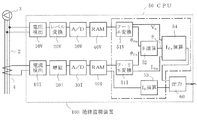•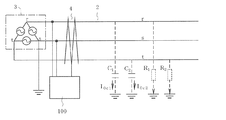•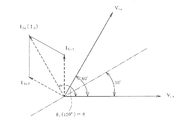•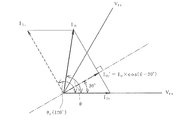•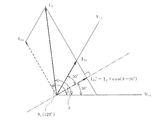•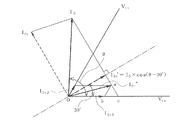••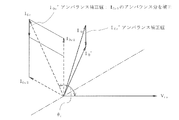•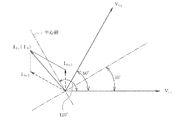•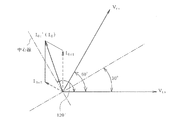•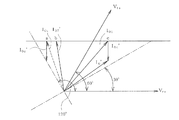## Description

なお、電路充電部から大地に流れる電流に関する定義としては、JIS C 8201-2-2「低圧開閉装置及び制御装置−第２−２部：漏電遮断器」が知られている。このため、本件出願では、このJIS C 8201-2-2を参考として以下の定義を用いることとする。
（１）地絡電流：絶縁不良に起因して、電路充電部から対地絶縁抵抗を介して大地に流れる電流（後述するように、記号Ｉ０ｒと表記する。）
（２）漏洩電流：電路充電部から対地静電容量を介して大地に流れる電流（記号Ｉ０ｃと表記する。）
（３）漏電電流：上記（１）の地絡電流と（２）の漏洩電流とのベクトル和（記号Ｉと表記する。）
The present invention relates to a ground fault current detection method and a detection device for detecting a ground fault current caused by a fault in ground insulation resistance (hereinafter also simply referred to as faulty insulation) from a leakage current of a three-phase power distribution system, and more particularly to a three-phase power distribution. The present invention relates to a technique suitable for application to a system insulation monitoring device, a leakage relay, or the like.
In addition, JIS C 8201-2-2 “Low voltage switchgear and control device—Part 2-2: Earth leakage breaker” is known as a definition related to the current flowing from the electric circuit charging unit to the ground. Therefore, in this application, the following definitions will be used with reference to JIS C 8201-2-2.
(1) Ground fault current: Current that flows from the current path charging section to the ground via the ground insulation resistance due to poor insulation (denoted as symbol I 0r as described later).
(2) Leakage current: Current that flows from the electric circuit charging unit to the ground via the ground capacitance (denoted as I0c )
(3) Leakage current: Vector sum of the ground fault current of (1) and the leakage current of (2) (denoted as symbol I 0 ).

As conventional techniques for monitoring insulation by measuring a ground fault current or ground insulation resistance of an AC distribution system, those described in Patent Documents 1 and 2 are known.
For example, in Patent Document 1, a first frequency component (for example, a fundamental wave component) and a second frequency component (for example, a fifth harmonic component) are extracted from the leakage current of the distribution system, and the second frequency component is a voltage. By converting to a value when the second frequency component is equal to the first frequency component, the ground fault current value caused by the ground insulation resistance is equal to the first frequency component and the second frequency component. Using the fact that the leakage current value caused by the difference in magnitude in proportion to the first frequency component and the second frequency component, the vector phase of the leakage current and the ground fault current is obtained respectively, and the ground insulation resistance An insulation monitoring device is disclosed in which a first frequency component, that is, a fundamental component of a ground fault current (in the three-phase grounding circuit, a current value of a vector sum of two-phase ground fault currents) is disclosed.
Patent Document 2 utilizes the fact that two types of 3n (n is an integer) harmonic component of the voltage and current of the distribution system are extracted, and that these 3n harmonic components are in phase with all three phases. In addition, a resistance measuring method or a monitoring device for obtaining the resistance component, that is, the ground insulation resistance value by solving simultaneous equations relating to the admittance of each harmonic component including the resistance component and the capacitance component is disclosed.
また、他の従来技術として、三相配電系統において各相の対地静電容量がバランスしているという仮定のもとで、測定した漏電電流から対地絶縁抵抗の劣化による地絡電流を計算するものがある。

Another conventional technique is to calculate the ground fault current due to the deterioration of the ground insulation resistance from the measured leakage current under the assumption that the ground capacitance of each phase is balanced in a three-phase distribution system. There is.
For example, in Patent Document 3, in the case where a ground fault current is detected based on a leakage current flowing in a ground line and a one-phase reference voltage that is not a ground phase, grounding is performed in one or both of two phases excluding the ground phase. An insulation monitoring device is disclosed in which a ground fault current is calculated by shifting the phase of the reference voltage by a predetermined angle so that an insulation state can be detected with a desired accuracy even when a fault current occurs.
In Patent Document 4, the ground fault current due to the deterioration of the ground insulation resistance is calculated from the phase angle and effective value of the measured leakage current, and the ground insulation resistance value is calculated using the ground fault current and the separately calculated voltage effective value. There is disclosed a leakage current interrupting device that calculates and interrupts an electric circuit to be measured when the ground fault current exceeds a predetermined value.

また、特許文献３及び特許文献４に係る従来技術は、何れも配電系統の対地静電容量がバランスしていることを前提としており、対地静電容量にアンバランスがある電路では、地絡電流の演算値に誤差が生じるという問題がある。
The prior arts related to Patent Document 1 and Patent Document 2 directly calculate a ground fault current from a leakage current by vector synthesis by a combination calculation of a fundamental wave component and a harmonic component, or two types of harmonic components, It is shown that the present invention can also be applied when the ground capacitance of the distribution system is unbalanced. However, in order to calculate the ground fault current by vector synthesis, generally complicated calculation processing is required.
The prior arts related to Patent Document 3 and Patent Document 4 are all premised on the fact that the ground capacitance of the distribution system is balanced, and in the electric circuit where the ground capacitance is unbalanced, the ground fault current There is a problem that an error occurs in the calculated value.
そこで、本発明の解決課題は、配電系統の対地静電容量がアンバランスの場合にも、対地絶縁抵抗の劣化に起因する地絡電流を高精度かつ容易に算出可能とした地絡電流検出方法及び検出装置を提供することにある。   Therefore, a problem to be solved by the present invention is to provide a ground fault current detection method capable of easily and accurately calculating a ground fault current due to deterioration of ground insulation resistance even when the ground capacitance of the distribution system is unbalanced. And providing a detection device.

In order to solve the above-described problem, a ground fault current detection method according to claim 1 is directed to a three-phase power distribution system having a three-phase delta-connected AC power source and one phase grounded. In a ground fault current detection method for detecting a ground fault current due to a ground insulation resistance of the distribution system using a current and a phase angle with respect to a line voltage of the leakage current,
Means for correcting the unbalance of the leakage current fundamental wave component by the harmonic component of the leakage current caused by the unbalance of the electrostatic capacitance to the ground of the distribution system or by the combination of the harmonic component and the fundamental wave component of the leakage current When the harmonic component is the nth (n is an odd number of 3 or more) harmonic component, the nth harmonic component of the line voltage is set to 1 / n of the fundamental wave component of the line voltage. Means for correcting an unbalance of the leakage current fundamental wave component using a value of the nth harmonic component of the leakage current when
The process of calculating the ground fault current with the phase angle for the line voltage fundamental component of the leakage current fundamental wave component of the leakage current fundamental component Toko obtained by correcting the imbalance, per a plurality of types of the n harmonic components This is executed to obtain a plurality of ground fault currents, and one of them is selected .

A ground fault current detection device according to a third aspect of the present invention is directed to a three-phase distribution system having a three-phase delta-connected AC power source and one phase of which is grounded, and the leakage current of the distribution system and the leakage current In the ground fault current detection device that calculates the ground fault current due to the ground insulation resistance of the distribution system using the phase angle with respect to the line voltage,
Means for detecting a leakage current of the distribution system; means for detecting a line voltage of the distribution system; means for extracting a fundamental wave component and a harmonic component from the leakage current and the line voltage; and Means for compensating a signal attenuated by an internal filter of the ground fault current detection device when extracting a fundamental wave component and a harmonic component from a line voltage according to the order of the harmonic component; and the leakage current By means of detecting the phase angle with respect to the line voltage and the harmonic component of the leakage current due to the unbalance of the ground capacitance of the distribution system, or by the combination of the harmonic component and the fundamental component of the leakage current , Correcting the unbalance of the leakage current fundamental wave component, correcting the imbalance, and the phase angle of the leakage current fundamental wave component with respect to the line voltage fundamental wave component, And means for calculating the ground fault current using those having a.

According to the present invention, it is possible to extract and detect only the ground fault current flowing to the ground due to insulation failure from the leakage current of the three-phase power distribution system. In particular, even when there is an unbalance in the ground capacitance of each phase, the ground fault current can be calculated with high accuracy using the leakage current and the phase angle corrected for this unbalance.
Furthermore, by obtaining the ground fault current as a scalar sum, the scalar sum of the ground fault current due to the ground insulation resistance is obtained in the same way as a conventional DC insulation resistance meter. A ground fault current similar to the meter can be detected.
In addition, the calculation process for obtaining the ground fault current is relatively simple, and there is an effect that the calculation load can be reduced.

Hereinafter, embodiments of the present invention will be described with reference to the drawings.
FIG. 1 is a configuration diagram when the ground fault current detection method and detection device of the present invention are applied to an insulation monitoring device 100. In FIG. 1, reference numeral 2 denotes a three-phase power distribution system having a three-phase delta-connected AC power supply 3, and the insulation monitoring device 100 detects a ground fault current flowing to the ground due to deterioration of the ground insulation resistance of the power distribution system 2. Is.

The line voltage of the distribution system 2 is input to the voltage detection means 10V in the insulation monitoring device 100, and the leakage current of the distribution system 2 detected by the zero phase current transformer (ZCT) 4 is supplied to the current detection means 10I. Have been entered. As described above, the leakage current is a vector sum of the leakage current and the ground fault current. Instead of the zero-phase current transformer 4, a current leakage current can be detected by connecting a normal current transformer (CT) to the ground line of a transformer (not shown) connected to the AC power source 3. good.
The voltage detection means 10V detects the line voltage of the distribution system 2 as a reference voltage, and the current detection means 10I is for detecting a leakage current (zero-phase current) I 0 flowing through the distribution system 2, It is assumed that the inter-voltage and the leakage current both include harmonic components in addition to the fundamental component of the system voltage.
レベル変換手段２０Ｖは、検出した線間電圧を後続の電子回路により処理可能な電圧レベルに変換し、また、増幅手段２０Ｉは、同じく零相変流器４の二次電流を電子回路により処理可能な電圧レベルに変換するためのものである。
レベル変換手段２０Ｖ及び増幅手段２０Ｉの各アナログ出力電圧は、Ａ／Ｄ変換手段３０Ｖ，３０Ｉにおいてそれぞれ一定周期でサンプリングされ、ディジタルデータに変換されてＲＡＭ等のメモリ４０Ｖ，４０Ｉにそれぞれ記憶される。メモリ４０Ｖ，４０Ｉに記憶される電圧データ、電流データの数としては、後述するフーリエ変換処理に十分な数、例えば系統電圧の１周期について３２個、６４個等が考えられるが、特に限定されるものではない。
The level conversion means 20V converts the detected line voltage into a voltage level that can be processed by the subsequent electronic circuit, and the amplification means 20I can also process the secondary current of the zero-phase current transformer 4 by the electronic circuit. It is for converting to a different voltage level.
The analog output voltages of the level converting means 20V and the amplifying means 20I are sampled at fixed periods by the A / D converting means 30V and 30I, converted into digital data, and stored in the memories 40V and 40I such as RAM. The number of voltage data and current data stored in the memories 40V and 40I may be a number sufficient for Fourier transform processing described later, for example, 32 or 64 for one period of the system voltage, but is particularly limited. It is not a thing.
また、５０は演算処理手段としてのＣＰＵであり、前記メモリ４０Ｖ，４０Ｉにそれぞれ記憶された電圧データ、電流データを用いて、以下の各手段が所定のプログラムに従って演算処理を実行するものである。   Reference numeral 50 denotes a CPU as arithmetic processing means, which uses the voltage data and current data respectively stored in the memories 40V and 40I to execute arithmetic processing according to a predetermined program.
まず、フーリエ変換手段５１Ｖは、メモリ４０Ｖ内の所定時間窓の電圧データを用いてフーリエ変換を行い、系統電圧の基本波成分及び高調波成分の振幅Ｖ及び絶対位相θＶｎ（ｎ＝１，３，５，７，・・・）を演算する。ここで、ｎ＝１は基本波成分を示し、ｎ＝３，５，７，・・・は各次の高調波成分を示す（以下、同じ）。
また、フーリエ変換手段５１Ｉは、メモリ４０Ｉ内の所定時間窓の電流データを用いてフーリエ変換を行い、上記と同様の原理に従って漏電電流Ｉの基本波成分及び高調波成分の振幅Ｉ０ｎ及び絶対位相θＩｎ（ｎ＝１，３，５，７，・・・）を演算する。

First, the Fourier transform means 51V performs Fourier transform using voltage data of a predetermined time window in the memory 40V, and the amplitude V n and the absolute phase θ Vn (n = 1, 1) of the fundamental wave component and the harmonic component of the system voltage. 3, 5, 7, ...). Here, n = 1 indicates the fundamental wave component, and n = 3, 5, 7,... Indicates the respective harmonic components (hereinafter the same).
Further, the Fourier transform means 51I performs Fourier transform using the current data of a predetermined time window in the memory 40I, and according to the same principle as described above, the amplitude I 0n and the absolute value of the fundamental wave component and the harmonic component of the leakage current I 0 The phase θ In (n = 1, 3, 5, 7,...) Is calculated.
For the Fourier transform, for example, either discrete Fourier transform (DFT) or fast Fourier transform (FFT) may be used. Techniques for obtaining the amplitude and absolute phase of fundamental and harmonic components of voltage and current by Fourier transform in this way are well known as described in, for example, Japanese Patent Application Laid-Open No. 2004-198273 and Japanese Patent No. 3599157. Therefore, detailed description is omitted here.

そして、地絡電流演算手段５４は、配電系統２の対地静電容量（後述するように非接地二相の対地静電容量）がバランスしている場合には、前記Ｖ，Ｉ０ｎ及びθを用いて、配電系統２の対地絶縁抵抗による地絡電流ｌ０ｒを求める。
また、配電系統２の対地静電容量にアンバランスがある場合には、前記Ｖ，Ｉ０ｎ及びθを用いて、漏電電流Ｉの基本波成分に対して上記アンバランス分を補正し、対地静電容量がバランスした状態に補正した漏電電流Ｉの基本波成分の大きさと、線間電圧基本波成分に対する位相角とを用いて、対地絶縁抵抗の劣化による地絡電流ｌ０ｒをスカラー和として求める。そして、この地絡電流ｌ０ｒは出力手段６０に送られる。
なお、これらの地絡電流ｌ０ｒの詳細な演算方法については、後に詳述する。
Then, when the ground capacitance of the power distribution system 2 (ungrounded two-phase ground capacitance as will be described later) is balanced, the ground fault current calculating means 54 is configured such that the V n , I 0n and θ Is used to determine the ground fault current 10r due to the ground insulation resistance of the distribution system 2.
When the ground capacitance of the distribution system 2 is unbalanced, the unbalanced part is corrected with respect to the fundamental component of the leakage current I 0 using the V n , I 0n and θ, Using the magnitude of the fundamental wave component of the leakage current I 0 corrected to balance the ground capacitance and the phase angle with respect to the line voltage fundamental wave component, the ground fault current l 0r due to the degradation of the ground insulation resistance is scalarized . Find as sum. The ground fault current 10r is sent to the output means 60.
The detailed calculation method of these ground fault currents 10r will be described in detail later.

Based on the current data stored in the memory 40I, the leakage current calculation means 53 calculates an effective value of the leakage current I 0 based on the same principle as that of a leakage breaker conventionally used in a low voltage distribution facility, It is sent to the output means 60 together with the ground fault current 10r .
The output means 60 performs display output, alarm output, transmission output to a host device, and the like based on the input ground fault current I 0r and leakage current I 0 .
ここで、図２は、前記配電系統２の一相（一例としてｓ相）が接地されている場合の系統構成図であり、絶縁監視装置１００にはｒ相、ｓ相間の線間電圧Ｖｒｓが基準電圧として入力されているものとする。

Here, FIG. 2 is a system configuration diagram in the case where one phase of the power distribution system 2 (for example, the s phase) is grounded, and the insulation monitoring apparatus 100 includes the line voltage V rs between the r phase and the s phase. Is input as a reference voltage.
In FIG. 2, C 1 and C 2 are the ground capacitances between the r-phase and t-phase, which are non-grounded phases, and the ground, respectively, and when the ground capacitances are balanced, C 1 = C 2 is there. R 1 and R 2 indicate the insulation resistance (ground insulation resistance) between the r phase, the t phase, and the ground, respectively.

なお、本実施形態では、地絡電流Ｉ０ｒを、前述の特許文献１，特許文献２等に記載されているようなベクトル合成による電流絶対値でなく、スカラー量（スカラー和）として得るようにしている。すなわち、配電系統の絶縁測定を行う従来の直流絶縁抵抗計では、各線路の対地絶縁抵抗を介して大地に流れる電流のスカラー和から絶縁抵抗を求めているため、本実施形態によれば、ＣＰＵ５０内の地絡電流演算手段５４において従来の直流絶縁抵抗計と同一の演算処理により地絡電流（言い換えれば絶縁抵抗）を演算することができると共に、活線状態のままで常時、絶縁監視を行うことを可能にしている。
The procedure for obtaining the ground fault current I 0r from the leakage current I 0 of the distribution system 2 under the above assumption will be described below.
In the present embodiment, the ground fault current I 0r is obtained as a scalar quantity (scalar sum) instead of the current absolute value by vector synthesis as described in Patent Document 1, Patent Document 2, and the like described above. ing. That is, in the conventional DC insulation resistance meter that performs insulation measurement of the distribution system, since the insulation resistance is obtained from the scalar sum of the currents flowing to the ground via the ground insulation resistance of each line, according to the present embodiment, the CPU 50 The ground fault current calculation means 54 can calculate the ground fault current (in other words, the insulation resistance) by the same calculation process as that of the conventional DC insulation resistance meter, and always performs insulation monitoring in the live line state. Making it possible.

また、漏洩電流Ｉ０ｃの位相角θは、線間電圧Ｖｒｓを基準としたときに１２０°の進み位相で一定である。漏電電流Ｉ（すなわち漏洩電流Ｉ０ｃ）の大きさと線間電圧Ｖｒｓに対する位相角θとを前述のフーリエ級数演算及び相対位相演算により求め、地絡電流演算手段５４が下記の数式１により地絡電流Ｉ０ｒを求めると、その値は前述のごとく零となる。
［数式１］
０ｒ＝Ｉ×ｃｏｓ（θ−３０°）×ｋ＝Ｉ０ｃ×ｃｏｓ（１２０°−３０°）×ｋ＝０
なお、ｋは、以下に述べるように、対地絶縁抵抗の劣化に起因して流れる地絡電流に応じた定数である。
Further, the phase angle θ c of the leakage current I 0c is constant at a leading phase of 120 ° when the line voltage V rs is used as a reference. The magnitude of the leakage current I 0 (that is, the leakage current I 0c ) and the phase angle θ c with respect to the line voltage V rs are obtained by the Fourier series calculation and the relative phase calculation, and the ground fault current calculation means 54 is calculated by the following formula 1. When the ground fault current I 0r is obtained, the value becomes zero as described above.
[Formula 1]
I 0r = I 0 × cos (θ−30 °) × k = I 0c × cos (120 ° −30 °) × k = 0
Note that k is a constant corresponding to the ground fault current that flows due to the deterioration of the ground insulation resistance, as described below.

Next, FIG. 4 is a vector diagram when the r-phase ground insulation resistance R 1 in FIG. 2 deteriorates and decreases, and a ground fault current I 0r flows.
Leakage current I 0 becomes a vector resultant value of the leakage current I 0c and ground fault current I 0r 4, the phase angle for the line voltage V rs of the leakage current I 0 becomes theta. Further, the phase angle of the leakage current I 0c becomes a leading phase of 120 ° with reference to the line voltage V rs as in FIG.
ここで、図４によれば、数式２、数式３が成立する。
［数式２］
０ｒ’＝Ｉ×ｃｏｓ（θ−３０°）
［数式３］
０ｒ’＝Ｉ０ｒ×ｃｏｓ（３０°）＝Ｉ０ｒ×√３／２
よって、上記数式２、数式３から数式４が導かれる。
［数式４］
０ｒ＝Ｉ×ｃｏｓ（θ−３０°）×２／√３＝Ｉ×ｃｏｓ（θ−３０°）×ｋ
（ｋ＝２／√３）
Here, according to FIG. 4, Formula 2 and Formula 3 are established.
[Formula 2]
I 0r '= I 0 × cos (θ-30 °)
[Formula 3]
I 0r ′ = I 0r × cos (30 °) = I 0r × √3 / 2
Therefore, Equation 4 is derived from Equation 2 and Equation 3 above.
[Formula 4]
I 0r = I 0 × cos (θ−30 °) × 2 / √3 = I 0 × cos (θ−30 °) × k
(K = 2 / √3)

この場合も、漏電電流Ｉは漏洩電流Ｉ０ｃと地絡電流Ｉ０ｒとのベクトル合成値となり、漏電電流Ｉの線間電圧Ｖｒｓに対する位相角はθとなる。また、対地静電容量に起因する漏洩電流Ｉ０ｃは、前記同様に線間電圧Ｖｒｓに対して１２０°の進み位相となる。
5, reduces the ground insulation resistance R 2 of the t-phase is a vector diagram when the ground fault current I 0r flows.
Also in this case, the leakage current I 0 is a vector composite value of the leakage current I 0c and the ground fault current I 0r, and the phase angle of the leakage current I 0 with respect to the line voltage V rs is θ. Further, the leakage current I 0c caused by the ground capacitance has a leading phase of 120 ° with respect to the line voltage V rs as described above.

［数式５］
０ｒ’＝Ｉ×ｃｏｓ（θ−３０°）
［数式６］
０ｒ’＝Ｉ０ｒ×ｃｏｓ（３０°）＝Ｉ０ｒ×√３／２

［数式７］
０ｒ＝Ｉ×ｃｏｓ（θ−３０°）×２／√３＝Ｉ×ｃｏｓ（θ−３０°）×ｋ
（ｋ＝２／√３）
Further, in FIG. 5, similarly to Equations 2 and 3, Equations 5 and 6 are established.
[Formula 5]
I 0r '= I 0 × cos (θ-30 °)
[Formula 6]
I 0r ′ = I 0r × cos (30 °) = I 0r × √3 / 2
Therefore, Equation 7 is derived from Equation 5 and Equation 6 above.
[Formula 7]
I 0r = I 0 × cos (θ−30 °) × 2 / √3 = I 0 × cos (θ−30 °) × k
(K = 2 / √3)
よって、ｔ相の絶縁抵抗Ｒが劣化して地絡電流Ｉ０ｒが流れた場合でも、地絡電流演算手段５４は、数式１と同様の数式７の演算により、漏洩電流Ｉ０ｃの影響を受けずにこれを除去した形で地絡電流Ｉ０ｒを検出することができる。 Therefore, even when the t-phase insulation resistance R 2 deteriorates and the ground fault current I 0r flows, the ground fault current calculation means 54 determines the influence of the leakage current I 0c by the calculation of Formula 7 similar to Formula 1. The ground fault current I 0r can be detected in such a manner that it is removed without receiving it.

この場合も、漏電電流Ｉは漏洩電流Ｉ０ｃと地絡電流Ｉ０ｒ”との合成値であり、漏電電流Ｉの線間電圧Ｖｒｓに対する位相角はθとなる。また、対地静電容量に起因する漏洩電流Ｉ０ｃは、前記同様に線間電圧Ｖｒｓに対して１２０°の進み位相となる。
FIG. 6 is a vector diagram when the ground insulation resistances R 1 and R 2 of both the r-phase and the t-phase are deteriorated and the ground fault currents I 0r1 and I 0r2 flow. The ground fault current obtained by vector synthesis of I 0r1 and I 0r2 is shown as I 0r ″.
Also in this case, the leakage current I 0 is a composite value of the leakage current I 0c and the ground fault current I 0r ″, and the phase angle of the leakage current I 0 with respect to the line voltage V rs is θ. The leakage current I 0c resulting from the capacitance has a leading phase of 120 ° with respect to the line voltage V rs as described above.

［数式８］
０ｒ’＝Ｉ×ｃｏｓ（θ−３０°）
また、求める地絡電流Ｉ０ｒの大きさは、Ｉ０ｒ１とＩ０ｒ２とのスカラー和である。ここで、図６において、Δａｂｃは各内角が６０°の正三角形であるから、線分ａｂ，ｂｃの長さは等しく、地絡電流Ｉ０ｒ２の大きさは線分ａｂ（＝ｂｃ）の長さに等しいと共に、地絡電流Ｉ０ｒ１の大きさは線分０ｂの長さに等しい。従って、図６における０ｃ（＝０ｂ＋ｂｃ）は地絡電流Ｉ０ｒの大きさに等しいため、Ｉ０ｒ’に関して数式９が成立する。
［数式９］
０ｒ’＝０ｃ×ｃｏｓ（３０°）＝Ｉ０ｒ×ｃｏｓ（３０°）＝Ｉ０ｒ×√３／２
In FIG. 6, Expression 8 is established similarly to Expression 2 and Expression 5.
[Formula 8]
I 0r '= I 0 × cos (θ-30 °)
The magnitude of the ground fault current I 0r to be obtained is a scalar sum of I 0r1 and I 0r2 . Here, in FIG. 6, Δabc is an equilateral triangle having an inner angle of 60 °, so the lengths of the line segments ab and bc are equal, and the magnitude of the ground fault current I 0r2 is the length of the line segment ab (= bc). And the magnitude of the ground fault current I 0r1 is equal to the length of the line segment 0b. Therefore, since 0c (= 0b + bc) in FIG. 6 is equal to the magnitude of the ground fault current I 0r , Equation 9 is established for I 0r ′.
[Formula 9]
I 0r '= 0c × cos (30 °) = I 0r × cos (30 °) = I 0r × √3 / 2

［数式１０］
０ｒ＝Ｉ×ｃｏｓ（θ−３０°）×２／√３＝Ｉ×ｃｏｓ（θ−３０°）×ｋ
（ｋ＝２／√３）

From Equation 8 and Equation 9, Equation 10 that is the same as Equation 1, Equation 4, and Equation 7 is derived as follows.
[Formula 10]
I 0r = I 0 × cos (θ−30 °) × 2 / √3 = I 0 × cos (θ−30 °) × k
(K = 2 / √3)
As can be understood from the above formulas 1, 4, 7, and 10 being the same calculation formula, the ground capacitance of the distribution system 2 (the ungrounded r-phase and t-phase ground capacitances C) 1 , C 2 ) are balanced, no matter what the values of the ground fault currents I 0r1 , I 0r2 due to the ground insulation resistances of the r-phase and t-phase are, The magnitude of the ground fault current I 0r is the same as the scalar quantity (scalar sum) based on the leakage current I 0 and the phase angle θ output from the Fourier transform means 51I and the relative phase calculation means 52 in FIG. The calculation algorithm can be used without any theoretical error.

As described above, when the ground capacitance of the distribution system 2 is balanced, the ground fault current I 0r can be obtained from the leakage current I 0 and the phase angle θ. Even in the case of balance, if the leakage current I 0 ′ corrected for the unbalance and the phase angle can be obtained , the magnitude of the ground fault current I 0r can be obtained as a scalar quantity by the same procedure as described above. it can.
In the following description, the leakage current I 0 ′ obtained by correcting the above-mentioned imbalance from the leakage current I 0c when the ungrounded r-phase and t-phase ground capacitances C 1 and C 2 are unbalanced and its leakage current I 0 ′ A method of obtaining the phase angle and calculating the magnitude of the ground fault current I 0r based on these will be described.
なお、対地静電容量のアンバランスは漏洩電流Ｉ０ｃに影響を及ぼし、ひいては漏電電流Ｉの大きさ及び位相角に影響するので、地絡電流Ｉ０ｒを高精度に検出するためには、上記アンバランスを補正した漏電電流Ｉ’を求めて地絡電流Ｉ０ｒを演算することが必要であり、以下の説明はこのような原理に基づくものである。また、以下では、線間電圧、漏電電流に含まれる高調波成分に着目したアンバランス補正値を用いて漏電電流Ｉの基本波成分を補正することにより、漏電電流Ｉ’を求めている。 In addition, since the unbalance of the ground capacitance affects the leakage current I 0c and thus affects the magnitude and phase angle of the leakage current I 0 , in order to detect the ground fault current I 0r with high accuracy, It is necessary to calculate the ground fault current I 0r by obtaining the leakage current I 0 ′ corrected for the unbalance, and the following explanation is based on such a principle. In the following, the leakage current I 0 ′ is obtained by correcting the fundamental wave component of the leakage current I 0 using the unbalance correction value focusing on the harmonic component included in the line voltage and leakage current. .

まず、図７は、線間電圧（第３調波）、及び、対地静電容量に起因した漏洩電流（第３調波）のベクトル図である。図７に示すように、線間電圧（第３調波）Ｖｒｓ，Ｖｔｓの相互の位相角は１８０°となる。これは、図３では線間電圧Ｖｒｓ，Ｖｔｓの基本波成分相互の位相角は６０°であったが、基本波成分の位相角に相当する位相時間は第３調波では３倍になるため、線間電圧（第３調波）Ｖｒｓ，Ｖｔｓの相互の位相角は６０°の３倍、つまり１８０°となるためである。
7 and 8 are vector diagrams in the case where the third harmonic component is used as the harmonic component for obtaining the unbalance correction value.
First, FIG. 7 is a vector diagram of the line voltage (third harmonic) and the leakage current (third harmonic) due to the ground capacitance. As shown in FIG. 7, the mutual phase angle of the line voltages (third harmonics) V rs and V ts is 180 °. In FIG. 3, the phase angle between the fundamental wave components of the line voltages V rs and V ts is 60 °, but the phase time corresponding to the phase angle of the fundamental wave component is tripled in the third harmonic. Therefore , the mutual phase angle of the line voltages (third harmonics) V rs and V ts is three times 60 °, that is, 180 °.

FIG. 8 is a diagram for explaining a method of correcting the fundamental wave component of the leakage current I 0 using the leakage current (third harmonic) I 0c described above.
As described above, the leakage current (third harmonic) I 0c resulting from the unbalance of the capacitance to the ground can be obtained from FIG. Using the unbalance correction value I 0c ′ obtained by converting this leakage current (third harmonic) I 0c into an unbalanced component of the fundamental wave component of the leakage current I 0 , the fundamental wave component of the leakage current I 0 is corrected. Thus, the leakage current I 0 ′ when the ground capacitance is balanced can be obtained.
ここで、対地静電容量に起因する漏洩電流の大きさは周波数に比例するから、電圧が同一の場合には、漏洩電流（第３調波）の大きさは基本波成分の３倍となる。このことは、線間電圧の第３調波成分の大きさが基本波成分の１／３のときに、漏洩電流（第３調波）の大きさが基本波成分の大きさに等しくなることを意味している。
よって、漏洩電流（第３調波）Ｉ０ｃを漏電電流Ｉの基本波成分に換算するには、線間電圧の第３調波成分の大きさを基本波成分の１／３としたときの漏洩電流値をアンバランス補正値Ｉ０ｃ’とし、このアンバランス補正値Ｉ０ｃ’により漏電電流Ｉの基本波成分を補正して新たに漏電電流Ｉ’を求めれば良い。
すなわち、線間電圧Ｖｒｓの基本波成分をＶｒｓ１、第３調波成分をＶｒｓ３とし、漏洩電流（第３調波）をＩ０ｃとすれば、アンバランス補正値Ｉ０ｃ’は数式１１によって求められる。
［数式１１］
０ｃ’＝Ｉ０ｃ×（１／３）×（Ｖｒｓ１／Ｖｒｓ３
Here, since the magnitude of the leakage current caused by the ground capacitance is proportional to the frequency, when the voltage is the same, the magnitude of the leakage current (third harmonic) is three times the fundamental wave component. . This means that when the magnitude of the third harmonic component of the line voltage is 1/3 of the fundamental component, the magnitude of the leakage current (third harmonic) is equal to the magnitude of the fundamental component. Means.
Therefore, in order to convert the leakage current (third harmonic) I 0c into the fundamental wave component of the leakage current I 0 , the magnitude of the third harmonic component of the line voltage is 1/3 of the fundamental wave component. The leakage current value is set to an unbalance correction value I 0c ′, and the fundamental wave component of the leakage current I 0 is corrected by the unbalance correction value I 0c ′ to newly obtain the leakage current I 0 ′.
That is, assuming that the fundamental wave component of the line voltage V rs is V rs1 , the third harmonic component is V rs3 , and the leakage current (third harmonic) is I 0c , the unbalance correction value I 0c ′ is expressed by Equation 11 Sought by.
[Formula 11]
I 0c ′ = I 0c × (1/3) × (V rs1 / V rs3 )

［数式１２］
θ＝ｃｏｓ−１｛ｘ／√（ｘ＋ｙ）｝
As shown in FIG. 8, by correcting I 0 using the unbalance correction value I 0c ′ (adding I 0c ′ to I 0 as a vector), an earth leakage in which the unbalanced portion of the ground capacitance is corrected is corrected. The current I 0 ′ can be obtained. Since the magnitude and phase angle of I 0 and the magnitude and phase angle of the converted value I 0c ′ are fixed, the calculation of I 0 ′ is easy, and the phase angle θ is also a vector of I 0 ′. If the coordinates (x, y) are obtained, it is obvious that the equation (12) is obtained.
[Formula 12]
θ = cos −1 {x / √ (x 2 + y 2 )}
なお、図７では電流が対地静電容量成分のみを含む例を示したが、対地抵抗成分も含む場合には、漏電電流Ｉの第３調波成分とその位相角とから、９０°の進み位相成分または２７０°の進み位相成分を演算により求めれば漏洩電流（第３調波）Ｉ０ｃを求めることができ、以後は上記と同様の演算によって漏電電流Ｉ’を求めることが可能である。

Note that FIG. 7 shows an example in which the current includes only the ground capacitance component. However, in the case where the current also includes the ground resistance component, 90 ° is obtained from the third harmonic component of the leakage current I 0 and its phase angle. The leakage current (third harmonic) I 0c can be obtained by calculating the leading phase component or the leading phase component of 270 °, and thereafter the leakage current I 0 ′ can be determined by the same calculation as described above. is there.
As described above, even when the ground capacitance is unbalanced, the unbalance is corrected by the unbalance correction value I 0c ′ to obtain I 0 ′, and this I 0 ′ and the phase angle θ are used. Then, if the calculation of Equation 10 or the like is performed , the magnitude of the ground fault current I 0r can be obtained as a scalar quantity.

9 and 10 are vector diagrams in the case where the fifth harmonic component is used as the harmonic component for performing the unbalance correction.
The mutual phase angle of the fifth harmonic components of the line voltages V rs and V ts is a phase where V rs is advanced by 60 ° with respect to V ts . In FIG. 3, the fundamental wave component of the line voltage has a phase where V ts is advanced by 60 ° with respect to V rs , but the 60 ° phase time of the fundamental wave component has a phase angle of 5 times in the fifth harmonic and is 300 °. Is the leading phase. This is equivalent to V rs being a 60 ° advance phase with respect to V ts .

これらの図９、図１０を比較すると、対地静電容量に起因する漏洩電流の基本波成分及び第５調波成分Ｉ０ｃ（Ｉ）は、位相角が１２０°の中心線を基準として対称となっている。このことは、漏洩電流の第５調波成分を漏洩電流の基本波成分に等しい大きさに換算した上で両者をベクトル加算すれば、漏洩電流の位相角は中心線の位相角である１２０°になることを示している。そしてこの位相角（１２０°）は、対地静電容量Ｃ，Ｃがバランスしているときの漏洩電流の位相角に等しい。
FIG. 9 shows the vector phase relationship of the fundamental wave component of the leakage current caused by the ground capacitance when the t-phase ground capacitance C 2 is larger than the r-phase C 1 in FIG. It is based on rs . FIG. 10 shows the vector phase relationship of the fifth harmonic component of the same leakage current as described above, which is based on V ts .
Comparing FIG. 9 and FIG. 10, the fundamental wave component and the fifth harmonic component I 0c (I 0 ) of the leakage current caused by the ground capacitance are symmetrical with respect to the center line having a phase angle of 120 °. It has become. This means that if the fifth harmonic component of the leakage current is converted to a magnitude equal to the fundamental wave component of the leakage current and the vectors are added together, the phase angle of the leakage current is 120 ° which is the phase angle of the center line. It shows that it becomes. This phase angle (120 °) is equal to the phase angle of the leakage current when the ground capacitances C 1 and C 2 are balanced.

ここで、線間電圧Ｖｒｓの基本波成分をＶｒｓ１、第５調波成分をＶｒｓ５、漏洩電流の第５調波成分をＩ０ｃとすれば、アンバランス補正値Ｉ０ｃ’は数式１３によって求められる。
［数式１３］
０ｃ’＝Ｉ０ｃ×（１／５）×（Ｖｒｓ１／Ｖｒｓ５
In order to convert the fifth harmonic component of the leakage current into the same magnitude as the fundamental component of the leakage current, the leakage current caused by the capacitance is proportional to the frequency. The fifth harmonic component of the current is five times the fundamental component. This indicates that when the fifth harmonic component of the line voltage is 1/5 of the fundamental component, the fifth harmonic component of the leakage current is equal to the fundamental component. Therefore, the leakage current value when the fifth harmonic component of the line voltage is 1/5 of the fundamental wave component is set as the unbalance correction value I 0c ′, and the fifth harmonic component of the leakage current is equal to the fundamental wave component. Convert to size.
Here, if the fundamental wave component of the line voltage V rs is V rs1 , the fifth harmonic component is V rs5 , and the fifth harmonic component of the leakage current is I 0c , the unbalance correction value I 0c ′ is expressed by Equation 13: Sought by.
[Formula 13]
I 0c ′ = I 0c × (1/5) × (V rs1 / V rs5 )

なお、この場合の地絡電流Ｉ０ｒは基本波成分と第５調波成分との和であるため、第５調波成分を除去することにより基本波成分を求める必要がある。
From the above, the phase of the current obtained by vector addition of the fundamental wave component of the leakage current and the unbalance correction value I 0c ′ according to Equation 13 is 120 °, which is the phase angle of the center line in FIGS. This is the phase angle of the leakage current when the capacity is balanced. By using this leakage current as the leakage current I 0 and performing the calculation of Equation 10 and the like in the same manner as when the ground capacitance is balanced, the ground fault current I 0r can be obtained as a scalar quantity.
Since the ground fault current I 0r in this case is the sum of the fundamental wave component and the fifth harmonic component, it is necessary to obtain the fundamental wave component by removing the fifth harmonic component.

FIG. 11 is a vector diagram when the seventh harmonic component is used as the harmonic component for performing the unbalance correction.
The mutual phase angle of the seventh harmonic components of the line voltages V rs and V ts is a phase that V ts advances by 60 ° with respect to V rs . In FIG. 3, the fundamental wave component of the line voltage has a phase where V ts is advanced by 60 ° with respect to V rs , but the 60 ° phase time of the fundamental wave component has a phase angle that is 7 times that of the seventh harmonic, and is 420 ° Is the leading phase. When V rs is used as a reference, V ts advances by 60 ° with respect to V rs , and the same advance as the fundamental wave.

すなわち、線間電圧Ｖｒｓの基本波成分をＶｒｓ１、第７調波成分をＶｒｓ７、漏洩電流の第７調波成分をＩ０７とすれば、アンバランス補正値Ｉ０７’は数式１４によって求められる。
［数式１４］
０７’＝Ｉ０７×（１／７）×（Ｖｒｓ１／Ｖｒｓ７
このアンバランス補正値Ｉ０７’と漏電電流の基本波成分Ｉ０１とを比較すると、対地静電容量に起因する漏洩電流は等しく、対地絶縁抵抗に起因する地絡電流については、第７調波成分が基本波成分の１／７となる。
In order to convert the seventh harmonic component of the leakage current into a magnitude equal to the fundamental component of the leakage current, since the leakage current due to the ground capacitance is proportional to the frequency, when the line voltage is the same, The seventh harmonic component of the leakage current is seven times the fundamental wave component. This indicates that the seventh harmonic component of the leakage current is equal to the fundamental component when the seventh harmonic component of the line voltage is 1/7 of the fundamental component. Therefore, the leakage current value when the seventh harmonic component of the line voltage is 1/7 of the fundamental wave component is set as the unbalance correction value I 0c ′, and the seventh harmonic component of the leakage current is equal to the fundamental wave component. Convert to size.
That is, if the fundamental component of the line voltage V rs is V rs1 , the seventh harmonic component is V rs7 , and the seventh harmonic component of the leakage current is I 07 , the unbalance correction value I 07 ′ Desired.
[Formula 14]
I 07 ′ = I 07 × (1/7) × (V rs1 / V rs7 )
When this unbalance correction value I 07 ′ is compared with the fundamental wave component I 01 of the leakage current, the leakage current caused by the ground capacitance is equal, and the ground fault current caused by the ground insulation resistance is the seventh harmonic. The component is 1/7 of the fundamental wave component.
ここで、対地静電容量に起因する漏洩電流をＩ０ｃとし、Ｉ０ｃ，Ｉ０７’及びＩ０１のベクトルの先端を図１１に示すように各々ａ，ｂ，ｃとすれば、線分ａｂ，ａｃの長さは対地絶縁抵抗に起因した地絡電流に比例することにより、電圧に比例し、ａｂ：ａｃは１：７となる。この関係とＩ０１，Ｉ０７’の位置とにより、対地静電容量に起因する漏洩電流Ｉ０ｃのベクトル位置が求められる。これを対地静電容量がバランスしたときの値に補正するためには、Ｉ０ｃの位相角が１２０°となるようにＩ０ｃ’を求めれば良い。

Here, if the leakage current resulting from the ground capacitance is I 0c and the leading ends of the vectors I 0c , I 07 ′ and I 01 are a, b and c as shown in FIG. , Ac is proportional to the voltage by being proportional to the ground fault current caused by the ground insulation resistance, and ab: ac is 1: 7. Based on this relationship and the positions of I 01 and I 07 ′, the vector position of the leakage current I 0c caused by the ground capacitance is obtained. In order to correct this to a value when the ground capacitance is balanced, I 0c ′ may be obtained so that the phase angle of I 0c is 120 °.
Also for the fundamental wave component of the leakage current, if I 01 is corrected by the above I 0c ′, the leakage current I 0 ′ when the ground capacitance is balanced can be obtained. The ground fault current I 0r can be obtained as a scalar quantity by performing the calculation of Equation 10 or the like with the leakage current I 0 ′ in the same manner as when the above-mentioned ground capacitance is balanced.

このため、地絡電流検出装置としては、前述した各高調波成分に対する演算機能を図１のＣＰＵ５０が備えると共に、各高調波成分に対する複数の演算結果の中から最適の地絡電流を唯一決定するような手段を備えることが望ましい。このような手段の例としては、例えば含有率が最も大きい高調波成分に対する演算結果（地絡電流値）を選定する手段や、すべての高調波成分に対する演算結果の平均値を求める手段等が考えられるが、勿論、これらに限定されるものではない。
In the above embodiment, the method of obtaining the ground fault current I 0r by combining the fundamental component of voltage and current with the third harmonic component, the fifth harmonic component or the seventh harmonic component has been described. , The content rate of each harmonic component such as the third, fifth, seventh, etc., and the presence or absence of inclusion differ depending on the type of power supply or load, and not all harmonic components are necessarily contained.
For this reason, as the ground fault current detection device, the CPU 50 of FIG. 1 has the calculation function for each harmonic component described above, and the optimum ground fault current is uniquely determined from a plurality of calculation results for each harmonic component. It is desirable to provide such means. Examples of such means include means for selecting a calculation result (ground fault current value) for a harmonic component having the largest content rate, means for obtaining an average value of calculation results for all harmonic components, and the like. Of course, the present invention is not limited to these.
また、本実施形態では、上述のように第３調波成分または第５調波成分または第７調波成分を用いているが、これら以外の高調波成分を用いて対地静電容量のアンバランスを補正しても良いのは言うまでもない。

このことから、電子機器の内部フィルタによる信号の減衰分を各高調波に応じた補正係数にて補償すれば、元の信号に含有された各高調波成分を正確に再現することができ、最終的な地絡電流の演算精度を一層向上させることができる。
In the present embodiment, as described above, the third harmonic component, the fifth harmonic component, or the seventh harmonic component is used. However, the unbalanced capacitance of the ground using other harmonic components. Needless to say, it may be corrected.
Further, in an electronic device, it is conceivable that a high frequency noise signal is mixed in addition to a target signal. As a countermeasure, for example, a resistor and a capacitor are connected to the input unit such as the voltage detection unit 10V and the current detection unit 10I in FIG. In many cases, an R-C filter or the like is added. In this case, the higher-order harmonic component relative to the fundamental wave component, the greater the signal attenuation, but the attenuation ratio is always a constant value depending on the frequency.
Therefore, if the attenuation of the signal by the internal filter of the electronic device is compensated with a correction coefficient corresponding to each harmonic, each harmonic component contained in the original signal can be accurately reproduced, and finally It is possible to further improve the calculation accuracy of the ground fault current.

２：三相配電系統
３：交流電源
４：零相変流器
１０Ｖ：電圧検出手段
１０Ｉ：電流検出手段
２０Ｖ：レベル変換手段
２０Ｉ：増幅手段
３０Ｖ，３０Ｉ：Ａ／Ｄ変換手段
４０Ｖ，４０Ｉ：メモリ
５０：ＣＰＵ
５１Ｖ，５１Ｉ：フーリエ変換手段
５２：相対位相演算手段
５３：漏電電流演算手段
５４：地絡電流演算手段
６０：出力手段
１００：絶縁監視装置
2: Three-phase power distribution system 3: AC power supply 4: Zero-phase current transformer 10V: Voltage detection means 10I: Current detection means 20V: Level conversion means 20I: Amplification means 30V, 30I: A / D conversion means 40V, 40I: Memory 50: CPU
51V, 51I: Fourier transform means 52: Relative phase calculation means 53: Leakage current calculation means 54: Ground fault current calculation means 60: Output means 100: Insulation monitoring device

## Claims (3)

1. 三相デルタ結線の交流電源を有し、かつ一相が接地された三相配電系統を対象として、前記配電系統の漏電電流と、前記漏電電流の線間電圧に対する位相角とを用いて、前記配電系統の対地絶縁抵抗に起因した地絡電流を検出する地絡電流検出方法において、
前記配電系統の対地静電容量のアンバランスに起因する漏洩電流の高調波成分により、または、漏洩電流の高調波成分及び基本波成分の組み合わせにより、漏電電流基本波成分のアンバランスを補正する手段であって、前記高調波成分が第ｎ（ｎは３以上の奇数）調波成分であるときに、線間電圧の第ｎ調波成分を線間電圧の基本波成分の１／ｎとしたときの前記漏洩電流の第ｎ調波成分の値を用いて前記漏電電流基本波成分のアンバランスを補正する手段を備え、
前記アンバランスを補正した漏電電流基本波成分とこの漏電電流基本波成分の線間電圧基本波成分に対する位相角とを用いて地絡電流を演算する処理を、複数種類の第ｎ調波成分につき実行して複数の地絡電流を求め、そのうちの一つを選択することを特徴とする地絡電流検出方法。
For a three-phase power distribution system having a three-phase delta-connected AC power source and one phase grounded, using the leakage current of the distribution system and the phase angle of the leakage current with respect to the line voltage, In the ground fault current detection method for detecting the ground fault current due to the ground insulation resistance of the distribution system,
Means for correcting the unbalance of the leakage current fundamental wave component by the harmonic component of the leakage current caused by the unbalance of the electrostatic capacitance to the ground of the distribution system or by the combination of the harmonic component and the fundamental wave component of the leakage current When the harmonic component is the nth (n is an odd number of 3 or more) harmonic component, the nth harmonic component of the line voltage is set to 1 / n of the fundamental wave component of the line voltage. Means for correcting an unbalance of the leakage current fundamental wave component using a value of the nth harmonic component of the leakage current when
The process of calculating the ground fault current with the phase angle for the line voltage fundamental component of the leakage current fundamental wave component of the leakage current fundamental component Toko obtained by correcting the imbalance, per a plurality of types of the n harmonic components A ground fault current detection method comprising: executing a plurality of ground fault currents and selecting one of them .
2. 請求項１に記載した地絡電流検出方法において、
前記三相配電系統のうちの非接地二相の対地絶縁抵抗による地絡電流のスカラー和を演算することを特徴とする地絡電流検出方法。
In the ground fault current detection method according to claim 1,
Ground fault current detecting method characterized that you calculating the scalar sum of the ground fault current due to ground insulation resistance of an ungrounded two phases of the three-phase power distribution system.
3. 三相デルタ結線の交流電源を有し、かつ一相が接地された三相配電系統を対象として、前記配電系統の漏電電流と、前記漏電電流の線間電圧に対する位相角とを用いて、前記配電系統の対地絶縁抵抗による地絡電流を演算する地絡電流検出装置において、
前記配電系統の漏電電流を検出する手段と、
前記配電系統の線間電圧を検出する手段と、
前記漏電電流及び線間電圧から基本波成分及び高調波成分を抽出する手段と、
前記漏電電流及び線間電圧から基本波成分及び高調波成分を抽出する際に前記地絡電流検出装置の内部フィルタにより減衰した信号を、前記高調波成分の次数に応じて補償する手段と、
前記漏電電流の前記線間電圧に対する位相角を検出する手段と、
前記配電系統の対地静電容量のアンバランスに起因する前記漏洩電流の高調波成分により、または、漏洩電流の高調波成分及び基本波成分の組み合わせにより、漏電電流基本波成分のアンバランスを補正し、前記アンバランスを補正した漏電電流基本波成分と、この漏電電流基本波成分の線間電圧基本波成分に対する位相角とを用いて地絡電流を演算する手段と、
を備えたことを特徴とする地絡電流検出装置
For a three-phase power distribution system having a three-phase delta-connected AC power source and one phase grounded, using the leakage current of the distribution system and the phase angle of the leakage current with respect to the line voltage, in ground fault current detector for calculating a ground fault current due to ground insulation resistance of the distribution system,
Means for detecting a leakage current of the distribution system;
Means for detecting a line voltage of the distribution system;
Means for extracting a fundamental wave component and a harmonic component from the leakage current and the line voltage;
Means for compensating a signal attenuated by an internal filter of the ground fault current detection device when extracting a fundamental wave component and a harmonic component from the leakage current and a line voltage according to the order of the harmonic component;
Means for detecting a phase angle of the leakage current with respect to the line voltage;
Correct the unbalance of the leakage current fundamental wave component by the harmonic component of the leakage current due to the unbalance of the electrostatic capacitance to the distribution system or by the combination of the harmonic component and fundamental wave component of the leakage current. Means for calculating a ground fault current using the leakage current fundamental wave component corrected for the unbalance and the phase angle of the leakage current fundamental wave component with respect to the line voltage fundamental wave component;
Ground fault current detecting device characterized by comprising a.
JP2009205700A 2009-09-07 2009-09-07 Ground fault current detection method and detection apparatus Active JP5494929B2 (en)

## Priority Applications (1)

Application Number Priority Date Filing Date Title
JP2009205700A JP5494929B2 (en) 2009-09-07 2009-09-07 Ground fault current detection method and detection apparatus

## Applications Claiming Priority (1)

Application Number Priority Date Filing Date Title
JP2009205700A JP5494929B2 (en) 2009-09-07 2009-09-07 Ground fault current detection method and detection apparatus

## Publications (2)

Publication Number Publication Date
JP2011058826A JP2011058826A (en) 2011-03-24
JP5494929B2 true JP5494929B2 (en) 2014-05-21

# Family

## Family Applications (1)

Application Number Title Priority Date Filing Date
JP2009205700A Active JP5494929B2 (en) 2009-09-07 2009-09-07 Ground fault current detection method and detection apparatus

## Country Status (1)

JP (1) JP5494929B2 (en)

## Families Citing this family (4)

* Cited by examiner, † Cited by third party
Publication number Priority date Publication date Assignee Title
CN103995189B (en) * 2014-06-04 2017-01-04 国家电网公司 Three-phase condenser bank unbalance voltage loop check method
WO2016038651A1 (en) * 2014-09-08 2016-03-17 株式会社日立製作所 Insulation diagnosis system or rotating machine
JP6922816B2 (en) * 2018-03-29 2021-08-18 オムロン株式会社 Insulation measuring device and insulation measuring method
CN110133452B (en) * 2019-07-01 2020-03-10 华北电力大学 Mixed-voltage same-tower fault phase selection method based on waveform correlation coefficient and current mutation quantity

## Family Cites Families (4)

* Cited by examiner, † Cited by third party
Publication number Priority date Publication date Assignee Title
JPH0692995B2 (en) * 1992-04-24 1994-11-16 光商工株式会社 Insulation monitoring method in low piezoelectric path
JP4167872B2 (en) * 2001-10-04 2008-10-22 株式会社日立産機システム Leakage current monitoring device and monitoring system therefor
JP4143463B2 (en) * 2003-04-21 2008-09-03 三菱電機株式会社 Insulation monitoring device
JP4563144B2 (en) * 2004-11-11 2010-10-13 株式会社ネットインデックス Leakage current detection method and leak current detection device

## Also Published As

Publication number Publication date
JP2011058826A (en) 2011-03-24

## Similar Documents

Publication Publication Date Title
US8823307B2 (en) System for detecting internal winding faults of a synchronous generator, computer program product and method
US7999557B2 (en) Method for determining location of phase-to-earth fault
JP5672369B2 (en) Determination of fault current component of differential current
US8044666B2 (en) Method for determining location of phase-to earth fault
US10088546B2 (en) Method and apparatus to diagnose current sensor polarities and phase associations for a three-phase electric power system
JP4599120B2 (en) Electrical installation insulation monitoring device and method
US9829519B2 (en) Method and apparatus to commission voltage sensors and branch circuit current sensors for branch circuit monitoring systems
JP5494929B2 (en) Ground fault current detection method and detection apparatus
JP2013036884A (en) Insulation monitoring method and insulation monitor
JP4143463B2 (en) Insulation monitoring device
JP4993728B2 (en) Effective leakage current measuring instrument
US9897647B2 (en) Method and apparatus to commission voltage sensors and branch circuit current sensors for branch circuit monitoring systems
JP2007071774A (en) Insulation measuring method and apparatus therefor
JP4993727B2 (en) Leakage current measuring instrument
JP2011137718A (en) Device for monitoring high voltage insulation
JP5743296B1 (en) Leakage location exploration method and apparatus
JP2006010608A (en) Insulation level monitoring method for non-earthing electric line and device thereof
US20170331278A1 (en) Differential protection method and differential protection device for a transformer
KR101909379B1 (en) Leakage current measuring method and leakage current measuring apparatus
JP2009058235A (en) Leak current measuring instrument for electric path and electric apparatus, and its method
JP2008309681A (en) Insulation deterioration monitoring device and its method
JP2004242404A (en) Leakage detector
JP2011149959A (en) Insulation monitoring device
JP5501820B2 (en) Line characteristic calculation device and line characteristic calculation method
KR101276171B1 (en) insulation monitoring system

## Legal Events

Date Code Title Description
A625 Written request for application examination (by other person)

Free format text: JAPANESE INTERMEDIATE CODE: A625

Effective date: 20120809

A977 Report on retrieval

Free format text: JAPANESE INTERMEDIATE CODE: A971007

Effective date: 20131010

A131 Notification of reasons for refusal

Free format text: JAPANESE INTERMEDIATE CODE: A131

Effective date: 20131017

A521 Written amendment

Free format text: JAPANESE INTERMEDIATE CODE: A523

Effective date: 20131119

TRDD Decision of grant or rejection written
A01 Written decision to grant a patent or to grant a registration (utility model)

Free format text: JAPANESE INTERMEDIATE CODE: A01

Effective date: 20140205

A61 First payment of annual fees (during grant procedure)

Free format text: JAPANESE INTERMEDIATE CODE: A61

Effective date: 20140218

R150 Certificate of patent or registration of utility model

Ref document number: 5494929

Country of ref document: JP

Free format text: JAPANESE INTERMEDIATE CODE: R150

R250 Receipt of annual fees

Free format text: JAPANESE INTERMEDIATE CODE: R250

R250 Receipt of annual fees

Free format text: JAPANESE INTERMEDIATE CODE: R250

R250 Receipt of annual fees

Free format text: JAPANESE INTERMEDIATE CODE: R250

R250 Receipt of annual fees

Free format text: JAPANESE INTERMEDIATE CODE: R250

R250 Receipt of annual fees

Free format text: JAPANESE INTERMEDIATE CODE: R250